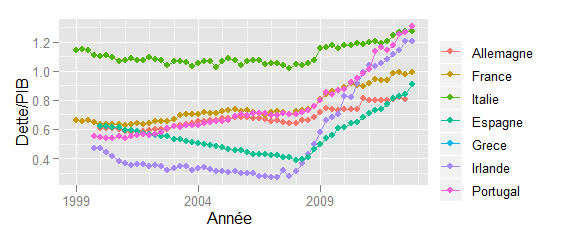[latexpage]In this post, I explain how to make two graphs from my post dealing with the article of Reinhart and Rogoff (french). The first figure is simple plot while the other is non parametric estimate by Splines.First of all,  you need the (obviously free) software R, once you’ve launched R, you need to install the ggplot2 package. Just run that code in the command window to install the package:

library(ggplot2)
setwd(‘C:/Users/Gauthier/Desktop/figures_RR/’)

This package is an easy and very nice plotting system. Download the data R&R I extracted from the OECD Database, I slightly reworked the dataset to load it easily in R. Edit this following command and set your own path to the folder where you have extracted your data,
setwd(‘C:/Users/Gauthier/Desktop/figures_RR/’)

Then we have to load the data via R. In the dataset, we have 3 time series of country members of the Euro Area: the public nominal debt, nominal GDP and the GDP deflator. To read them, you have to execute in the command windows,

# import value

First we must calculate the real variables: $GDP^{real}_{t}=GDP^{nominal}_{t}/Def_{t}$, $Debt^{real}_{t}=Debt^{nominal}_{t}/Def_{t}$ and the Debt to GDP ratio,

rdebt=debt/def
rgdp=gdp/def
ratio=rdebt/rgdp

Then we set our calculations in a data frame,

# convert to data frame
df
date=seq(as.Date(‘1999/1/1’), as.Date(‘2012/10/1’), by = ‘3 months’),
d = ratio
)

We can now start to plot the figures.

### Figure 1

We first declare a list of country we will plot, then we parse the list via a simple loop,

# plot fig 1
dt col=c(‘Allemagne’,’France’,’Italie’,’Espagne’,’Grece’,’Irlande’,’Espagne’,’Portugal’)#colnames(debt)
for( i in 1:length(col) ){
name=paste(‘d.’,col[i],sep = ”, collapse = NULL)
name2=paste(col[i])
eval(parse(text=paste(‘dt }
dt + xlab(‘Année’) + ylab(‘Dette/PIB’)

And we obtain the figure 1,### Figure 2

To draw the second figure that plots the correlation between the debt to GDP ratio with the GDP growth, we need to calculate the real GDP growth. We simply need to evaluate,
\begin{equation}\label{eq:MaxUtility}
\Delta GDP^{real}_{t}=\left(\frac{GDP^{real}_{t+1}}{GDP^{real}_{t}}-1\right)100
\end{equation}
We create the variable grgdp and we perform the calculation via another simple loop,

# computing real gdp growth
grgdp = rgdp[-dim(rgdp),]
for( t in 1:(dim(rgdp)-1) ){
grgdp[t,] = ((rgdp[t+1,]/rgdp[t,])-1)*100
}

And we set all these variables in a data frame,

x=c(as.matrix(ratio[-1,]))
y=c(as.matrix(grgdp))

myx=c()
myy=c()
for( i in 1:(length(x)) ){
if( is.na(x[i]) == FALSE && is.na(y[i]) == FALSE ){
myx=c(myx,x[i])
myy=c(myy,y[i])
}
}

mydata=data.frame(
growth=myy,
ratio=myx
)

We draw all the point by calling the function the geom_point(), then we use the function stat_smooth() to perform a non parametric estimation by splines with a 90% interval.

c <- ggplot(mydata, aes(ratio,growth)) + geom_point() + stat_smooth() + ylim(-2.5, 3) + xlab(‘Dette/PIB’) + ylab(‘Croissance PIB Réel’)
c

Then we obtain the figure 2.Get the whole code here.